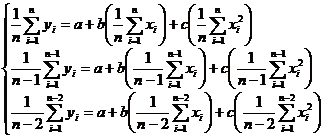§ 3    Arc method and average method of curve fitting

1. The arc method of curve fitting

Circular fitting is a geometric method that depicts a fitted curve through observation points ( model points ) .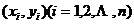It replaces curves with segmented arcs and makes two adjacent arcs have a common tangent. This approach boils down to the following three situations :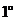Given circle O and two points outside the circle , , find the circle P , make it pass through the points , and be tangent ( circumscribed or inscribed ) with circle O ( Fig. 17.2 ) .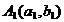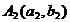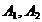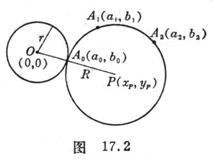Let the radius of the circle O be r and the coordinates of the point O to be ( 0,0 ) . remember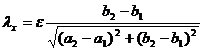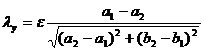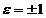, the symbol is inscribed or excised. remember again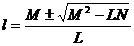in the formula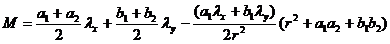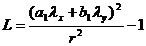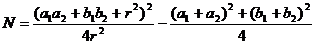then

( i ) The coordinates of the center of the circle P are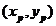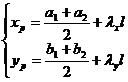( ii ) The radius R of the circle P is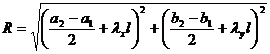( iii ) The coordinates of the tangent point are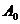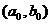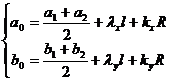in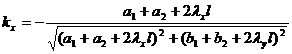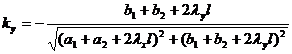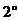Knowing the circle Q and a point outside the circle , find the circle P so that it passes through the fixed point and is tangent to the circle Q at the fixed point ( Figure 17.3 ) .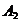Let the coordinates of the center of the circle Q be ( s, t ) , then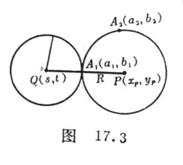( i ) The coordinates of the center of the circle P are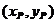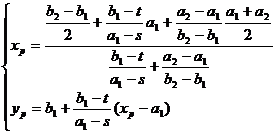( ii ) The radius R of the circle P is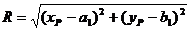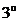Knowing the circle Q and the circle , find the circle P so that it is tangent to the circle and to the circle Q at a fixed point ( Fig. 17.4 ) .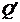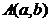Let the coordinates of the center of the circle Q be ( s, t ) and the radius be r ; the coordinates of the center of the circle are and the radius is . remember again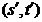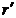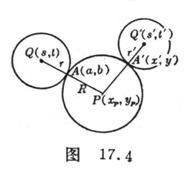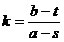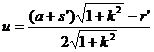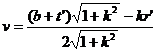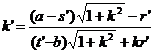then

( i ) The coordinates of the center of the circle P are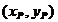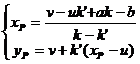( ii ) The radius R of the circle is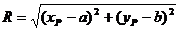( iii ) The coordinates ( x', y' ) of the tangent point A' are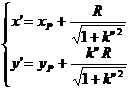in the formula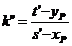Second, the average method of curve fitting

[ Linear ] If a series of data for two variables ( x, y ) are known to be

 x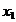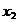…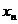y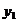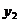…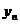Suppose x, y satisfy a linear relationship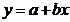Then a and b are determined by the following equations :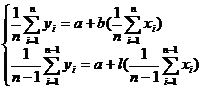The dispersion of the ordinate between the straight line obtained by this method and each point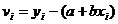The algebraic sum is zero.

[ Parabolic ]   If the straight line does not fit the trend of the known data, then the optional polynomial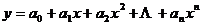to fit. For example, take the empirical curve as a quadratic polynomial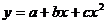a,b,c can be determined by the following three-dimensional linear equations :